Name:    Chapter 5 Test

Multiple Choice
Identify the choice that best completes the statement or answers the question.

The two triangles are similar. Find the measure of the angle.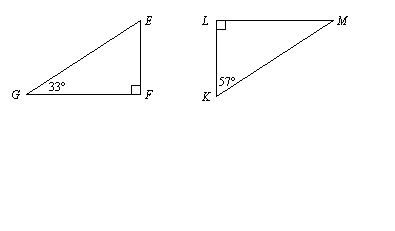1.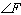a. 90° c. 57° b. 33° d. 180°

2.

In the figure,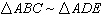.  Find the distance DE.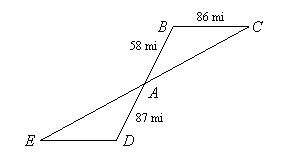a. 129 mi c. 124.1 mi b. 120.8 mi d. 57.3 mi

3.

A person standing 40 feet from a street light casts a shadow as shown. What is the height h of the street light? Assume the triangles are similar.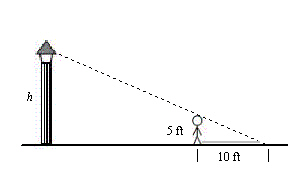a. 23 ft c. 27 ft b. 25 ft d. 50 ft

Use the map to find the actual distance between the cities.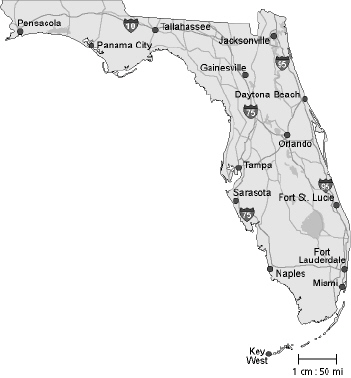4.

Naples and Miami
 a. 175 mi c. 100 mi b. 200 mi d. 50 mi

Find the missing dimension. Use the scale factor 1:12.

5.

 Item Model Actual Water Tower Height: 293 cm Height: __?__ m
 a. 3.516 m c. 3516 m b. 2441.667 m d. 35.16 m

Find the coordinates of the figure after reflecting in the y-axis.

6.

K(3, –3), L(8, –2), M(5, –6), N(0, –6)
 a. K'(3, –3), L'(8, –2), M'(5, –6), N'(0, –6) b. K'(–3, –3), L'(–8, –2), M'(–5, –6), N'(0, –6) c. K'(3, 3), L'(8, 2), M'(5, 6), N'(0, 6) d. K'(–3, 3), L'(–8, 2), M'(–5, 6), N'(0, 6)

A figure lies entirely in Quadrant II. In which quadrant will the figure lie after the given clockwise rotation about the origin?

7.

180°

The vertices of a parallelogram are B(–7, 1), C(–9, 5), D(–4, 5), and E(–2, 1). Rotate the parallelogram as described. Find the coordinates of the image.

8.

 a. B'(–7, –1), C'(–9, –5), D'(–4, –5), E'(–2, –1) b. B'(–1, –7), C'(–5, –9), D'(–5, –4), E'(–1, –2) c. B'(–1, 7), C'(–5, 9), D'(–5, 4), E'(–1, 2) d. B'(1, 7), C'(5, 9), D'(5, 4), E'(1, 2)

Numeric Response

1.

The ratio of the corresponding side lengths of two similar city blocks is 16:11. The perimeter of the larger block is 0.8 mile.  What is the perimeter of the smaller block?

2.

The ratio of the side length of Square A to the side length of Square B is 12:10. The side length of Square A is 18 centimeters. What is the perimeter of Square B?